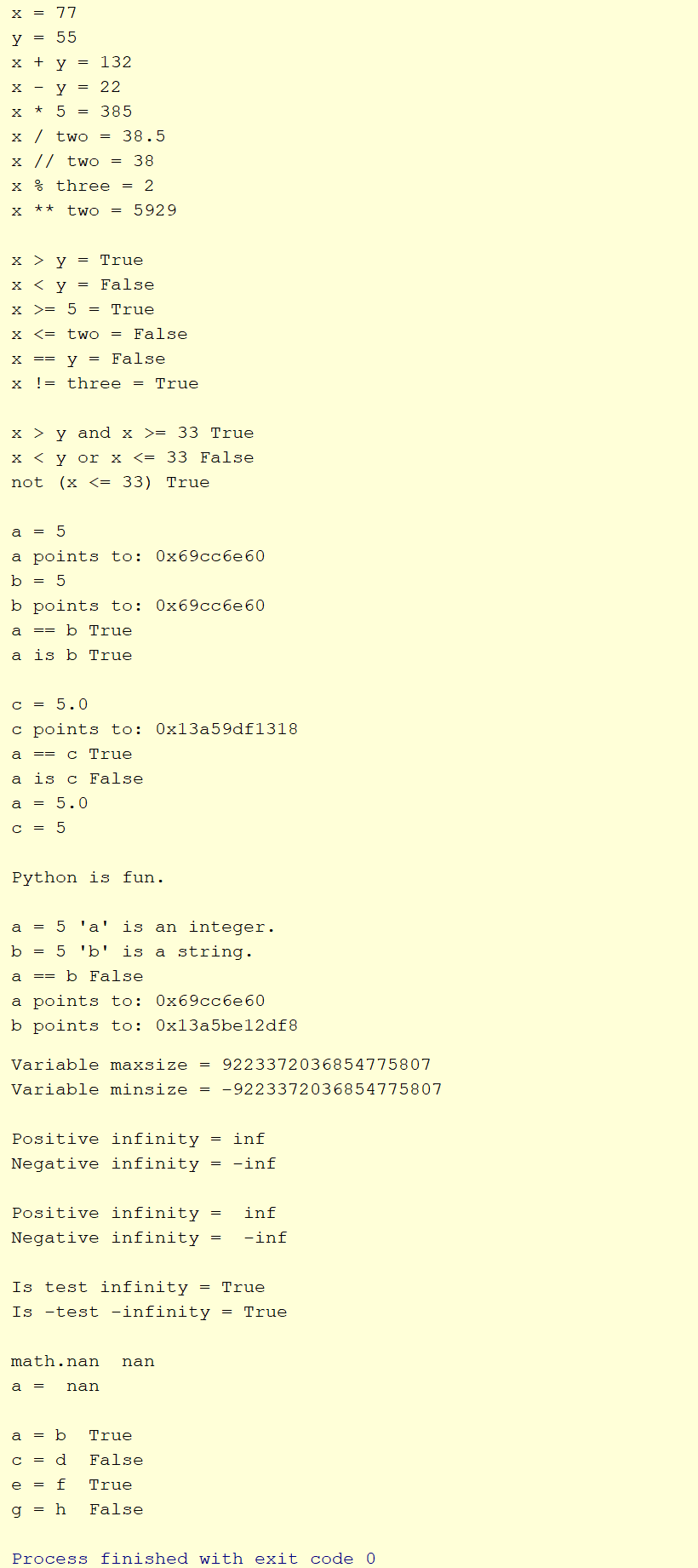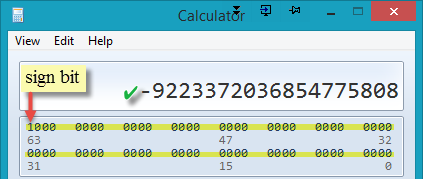# COSC 1336 | PyThon# Data Types

## Foreword

In this course, we will cover the most commonly used Python data types as outlined in the Python Language Reference. The following four general data types are discussed in the course and, unless otherwise stated, will be used in examples: Numbers (Integer, Boolean, Real, Complex), Sequences (Immutable - String, Tuple, Bytes; Mutable - Lists, Byte Arrays), Mappings (Dictionary), and Sets.

On occasion, the description of technical material can vary from document to document at python.org. On this site we defer to the content as described in the Python Language Reference as the authoritative source.## Data Types - Numbers

Numbers - numbers in Python come in the following forms: integral (integers and boolean), real (floating point numbers), and complex.

Integer numbers, specified by the built-in function 'int()', are simply whole numbers like 7, 482, -9993, etc.

Boolean has only two values, True and False. It is considered an integral type since the values True and False are internally analogous to 1 and 0 respectively.

Real numbers, specified by the built-in function 'float()', are numbers including a decimal like 33.89, 1.75364, -5226.88, etc.

Complex numbers have a 'real' and 'imaginary' part. They have practical applications in many physical scientific disciplines such as: biology, chemistry, electrical engineering, and physics. Complex numbers are beyond the scope of this course.

The mathematical operations in the table below can be used with the numbers data type.

 Mathematical Operations + Addition - Subtraction * Multiplication / division // Integer division (rounds toward zero) % Modulo ** Exponentiation

The relational operations in the table below can be used with the numbers data type.

 Relational Operations > Greater than < Less than >= Greater than or equal to <= Less or equal to == Equality != Not equal is true if same address

The logical operations in the table below can be used in simple and complex conditional statements. Logical operators are usually used in combination with relational operators as shown in the code example below.

 Logical Operations and returns True if two boolean expressions are both True or returns True if at least one boolean expression is True not reverses the value of a boolean expression

Lines 118 - 137 contain a few additional operations to consider related to the numbers data type. Positive Infinity and negative infinity are useful to test for extreme range values without 'guessing' or supplying artificially high/low numbers. NaN (not a number) is useful to determine if an operation returns a valid number. See this link for more on these topics.

Another common consideration for programmers is determining if two numbers are equal. With intergers, it is a simple test for equality. With real numbers however, to determine equality, we must consider precision. In this context, precision means the number of decimal places to compare in the test for equality. For example, is 7.00001 == 7.00000? The two numbers are 'close' but not equal if considering five or more digits to the right of the decimal. The library function math.isclose() can be used to compare real numbers. A few examples of math.isclose() are shown below. The argument 'rel_tol' can be set to modify the precision of the comparision. See this link for more on math.isclose().

The code below demonstrates each of the operations listed above.Here's the output. As always, ensure you understand the output based on the code example.The sys.maxsize() function is used to display the maximum variable size supported by Python. That number (9223372036854775807) can also be confirmed on the Windows calculator set to Programmer mode. Note that the value is 263 - 1. The reason it is not 264 is that one bit is reserved for the sign bit. So, Python accommodates a very large positive and a very large negative number as shown in the program output as max and min sizes. 263 = 9223372036854775808 but one number has to be reserved for '0' so it is 263 - 1 = 9223372036854775807 in the positive direction and -9223372036854775808. Positive numbers are represented with the sign bit set to '0' and negative numbers have the sign bit set to '1'.With the sign bit set to '1', the number will be negative. Notice that when all bits are set, the value is -1 which is the highest value negative integer. Note that when bit 63, the highest bit is set, the result will be a negative number.As bit are 'taken away' from the right, the negative number grows.Finally, the lowest value negative number is reached by setting all values to '0' except the sign bit. With the sign bit set to '1', the number will be negative.In the image below, notice with the calculator set to display hexadecimal numbers that 9223372036854775807 = 7FFFFFFFFFFFFFFF. Each of the four bits is called a nibble. Eight bits = one byte. There are a total of 64 bits used to represent a 'signed' variable on a 64-bit machine running a 64-bit operating system.